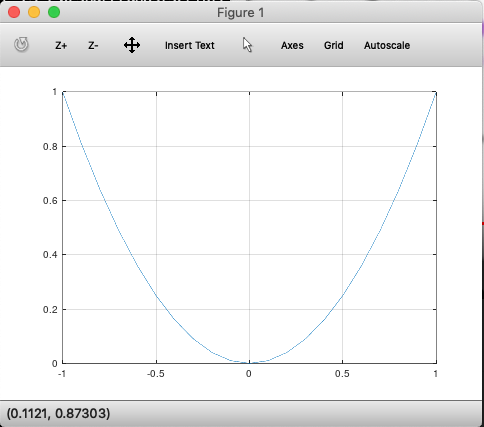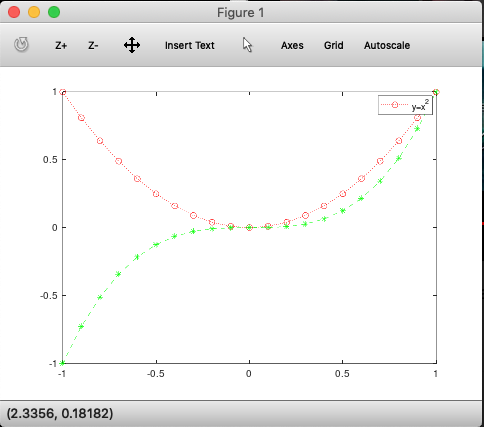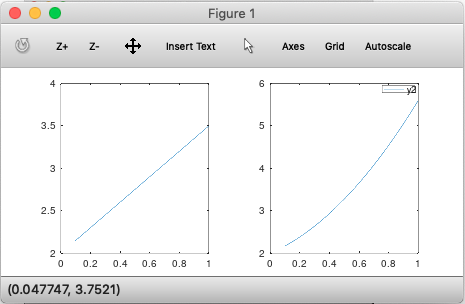# Handling and Plotting data in Octave / MATLAB

### What’s in memory¶

To quickly view the variables in memory, use the `who` command. Use `whos` for detailed view

```>> who
Variables in the current scope:
ans       ar1       heat      readings  vec       vec1      vec44     vec45     x         y         z

>> whos
Variables in the current scope:
Attr Name          Size                     Bytes  Class
==== ====          ====                     =====  =====
ans           1x78                        78  char
ar1           1x4                         32  double
heat          1x1                          8  double
vec           1x16                        24  double
vec1          1x4                         32  double
vec44         4x4                        128  double
vec45         4x5                        160  double
x             1x21                        24  double
y             1x21                       168  double
z             1x21                       168  double

Total is 218 elements using 950 bytes
```

To delete a variable from memory, use the `clear <variable name>` command. Just using the `clear` command will remove all variables.

Read datasets with `load` command. `>> load('ex1data1.txt')` A variable with the name of the file is created in memory. You can use array slicing, dicing to move a part of this data into a new variable.

```>> load('ex1data1.txt');
>> subset = ex1data1(1:10,:)
subset =
6.1101   17.5920
5.5277    9.1302
8.5186   13.6620
7.0032   11.8540
5.8598    6.8233
8.3829   11.8860
7.4764    4.3483
8.5781   12.0000
6.4862    6.5987
5.0546    3.8166
```

### Saving data to disk¶

Use `save <filename.extn> <variable> <-options>` to save a variable to disk.

```>> save 'subset.mat' subset
```

You can load this back into memory using the `load` command: `load('subset.mat')`. This time, since it is a `mat` file, Octave will load it with the original variable name.

To save data in a human readable form, use `save <filename.txt> <variable> -ascii`.

### Plotting¶

Most 2D plots can be accomplished using `plot(<arr1>, <arr2>, 'srt:options')` function.

#### Line plots¶

```plot(x,y); % will plot in a new window
```We can customize the appearance of ticks and line by passing them as a string. For instance, `r:*` will make lines in red, `*` for points and `:` for dotted lines.

You can also customize the title, labels, legend as shown:

```>> plot(x,y);
>> xlabel('time[x]');
>> ylabel('y=x^2');
>> legend('y(x)')
>> title('Function of time')
```

#### Overlaying plots¶

To overlay multiple plots on the same frame, use `hold on` command.

```>> z = x.^3;
>> plot(x,y, 'r:o')
>> hold on
>> plot(x,z, 'g--*')
```You can also plot multiple plots in the same command as `plot(x,y, x,z)` which will overlay both `y` and `z` on the same plot window.

To close the current figure, call the `close` command.

#### Printing plots to disk¶

To print to disk, use the `print` command as `print -dpng 'myplot.png'`. This will print the current plot to disk.

#### Multiple plot windows¶

Use the `figure(n)` command to create new plot windows:

```>> figure(1); plot(x,y1);
>> figure(2); plot(y1,y2);
```

will open 2 plot windows, one for each plot command.

#### Subplots¶

Use the `subplot(nrows, ncols, current_cell)` command to create and activate a plot window:

```>> subplot(1,2,1);
>> plot(x,y1);
>> axis([0,1]);  % Sets axis limits. Syntax is axis([xmin, xmax, ymin, ymax])
>> subplot(1,2,2);
>> plot(x,y2);
>> legend('y2');
```

which produces:#### Visualizing matrices as images¶

To quickly ‘see’ a matrix as a color coded image, use `imagesc`:

```>> x=randn(900,1);  % produces a standard normal dist, mean=0
>> size(x)
ans =
900     1
>> x2d= reshape(x,30,30); % turns this vector to a 2d matrix
>> imagesc(x2d);  % turns this matrix to an image
>> colorbar;  % adds a colorbar legend
```